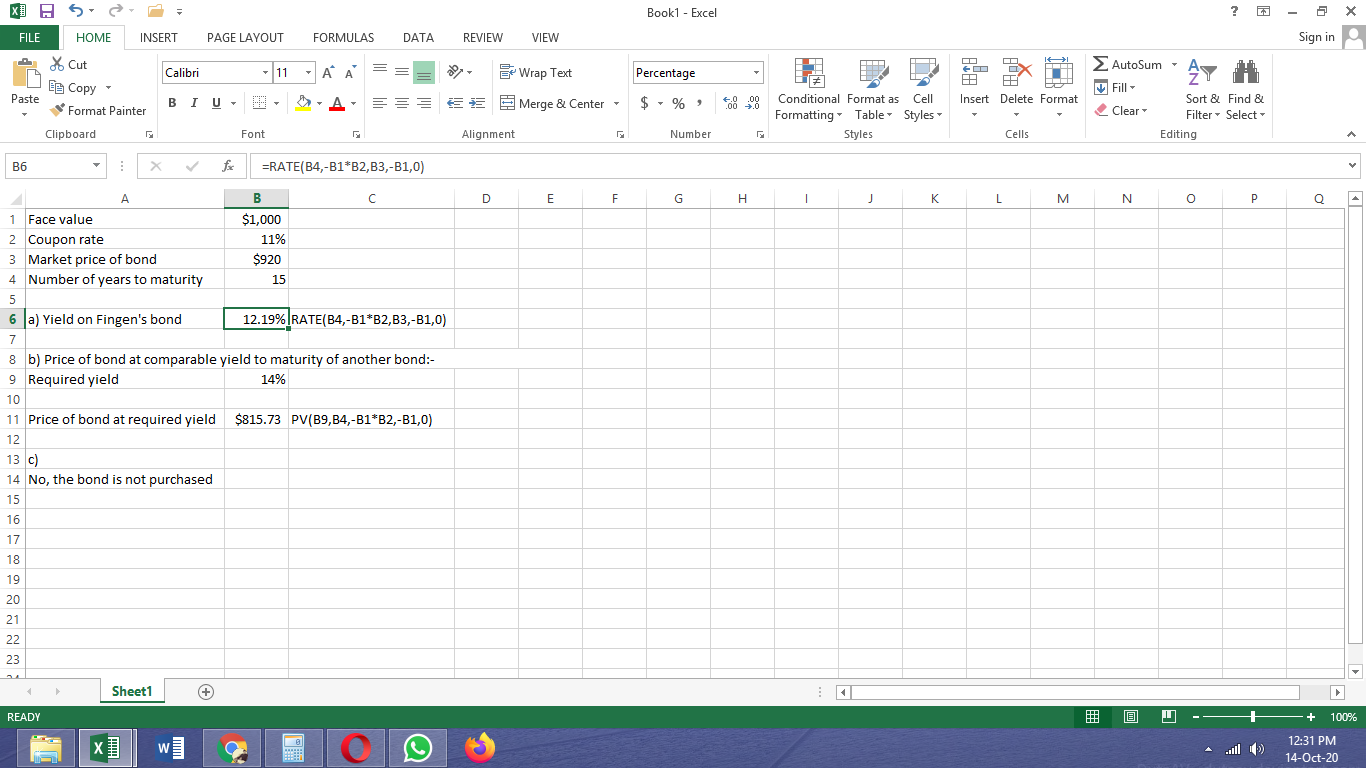In: Finance

# Fingen's 15​-year, ​$1,000 par value bonds pay 11 percent interest annually. The market price of the bonds... Fingen's 15​-year, ​$1,000  par value bonds pay 11 percent interest annually. The market price of the bonds is ​$920 and the​ market's required yield to maturity on a​ comparable-risk bond is 14 percent. a. Compute the​ bond's yield to maturity. b. Determine the value of the bond to​ you, given your required rate of return. c. Should you purchase the​ bond? ## Solutions ##### Expert Solution## Related Solutions ##### ​Fingen's 12​-year, ​$1,000 par value bonds pay 15 percent interest annually. The market price of the...
​Fingen's 12​-year, ​$1,000 par value bonds pay 15 percent interest annually. The market price of the bonds is ​$880 and the​ market's required yield to maturity on a​ comparable-risk bond is 16 percent. a.  Compute the​ bond's yield to maturity. b.  Determine the value of the bond to​ you, given your required rate of return. c.  Should you purchase the​ bond?
##### Fingen's 15​-year, ​$1,000 par value bonds pay 12 percent interest annually. The market price of the... Fingen's 15​-year, ​$1,000 par value bonds pay 12 percent interest annually. The market price of the bonds is ​$1,100 and the​ market's required yield to maturity on a​ comparable-risk bond is 9 percent. a. Compute the​ bond's yield to maturity. b. Determine the value of the bond to​ you, given your required rate of return. c. Should you purchase the​ bond? ##### ​Fingen's 13​-year, ​$1,000 par value bonds pay 9 percent interest annually. The market price of the...
​Fingen's 13​-year, ​$1,000 par value bonds pay 9 percent interest annually. The market price of the bonds is ​$850 and the​ market's required yield to maturity on a​ comparable-risk bond is 10 percent. a. Compute the​ bond's yield to maturity. b. Determine the value of the bond to​ you, given your required rate of return. c. Should you purchase the​ bond?
##### (Bond valuation)?Fingen's 14?-year, ?$1,000 par value bonds pay 13 percent interest annually. The market price of... (Bond valuation)?Fingen's 14?-year, ?$1,000 par value bonds pay 13 percent interest annually. The market price of the bonds is ?$870 and the? market's required yield to maturity on a? comparable-risk bond is 14 percent. a. Compute the? bond's yield to maturity. b. Determine the value of the bond to? you, given your required rate of return. c. Should you purchase the? bond? a. What is your yield to maturity on the Fingen bonds given the market price of the? bonds?... ##### ​(Bond valuation) ​Fingen's 16​-year,$1,000 par value bonds pay 14 percent interest annually. The market price...
​(Bond valuation) ​Fingen's 16​-year, $1,000 par value bonds pay 14 percent interest annually. The market price of the bonds is$870 and the​ market's required yield to maturity on a​ comparable-risk bond is 15 percent. a.  Compute the​ bond's yield to maturity. b.  Determine the value of the bond to​ you, given your required rate of return. c.  Should you purchase the​ bond? a.  What is your yield to maturity on the Fingen bonds given the market price of the​...
##### The 16​-year, ​$1,000 par value bonds of Waco Industries pay 11 percent interest annually. The market... The 16​-year, ​$1,000 par value bonds of Waco Industries pay 11 percent interest annually. The market price of the bond is ​$945​, and the​ market's required yield to maturity on a​ comparable-risk bond is 10 percent. a. Compute the​ bond's yield to maturity. b. Determine the value of the bond to you given the​ market's required yield to maturity on a​ comparable-risk bond. c. Should you purchase the​ bond? ##### The 11​-year, ​$1,000 par value bonds of Waco Industries pay 9 percent interest annually. The market...
The 11​-year, ​$1,000 par value bonds of Waco Industries pay 9 percent interest annually. The market price of the bond is ​$1,095​, and the​ market's required yield to maturity on a​ comparable-risk bond is 6 percent. a.  Compute the​ bond's yield to maturity. b.  Determine the value of the bond to you given the​ market's required yield to maturity on a​ comparable-risk bond. c.  Should you purchase the​ bond?
##### The 11-year, $1,000 par value bonds of Witt Industries pay 7 percent interest annually. The market... The 11-year,$1,000 par value bonds of Witt Industries pay 7 percent interest annually. The market price of the bond is $865, and the market’s required yield to maturity on a comparable-risk bond is 10 percent. A. What is your yield to maturity on the Witt bonds given the current market price of the bonds? _% Please round to two decimal places. B. What should be the value of the Witt bonds given the market's required yield to maturity on... ##### 7) Part 1. (Bond valuation)Fingen's 19?-year, ?$1,000 par value bonds pay 13 percent interest annually. The...
7) Part 1. (Bond valuation)Fingen's 19?-year, ?$1,000 par value bonds pay 13 percent interest annually. The market price of the bonds is ?$1,100 and the? market's required yield to maturity on a? comparable-risk bond is 10 percent. a. Compute the? bond's yield to maturity. b. Determine the value of the bond to? you, given your required rate of return. c. Should you purchase the? bond? Part 2. (Yield to? maturity) Abner? Corporation's bonds mature in 19 years and pay 11...